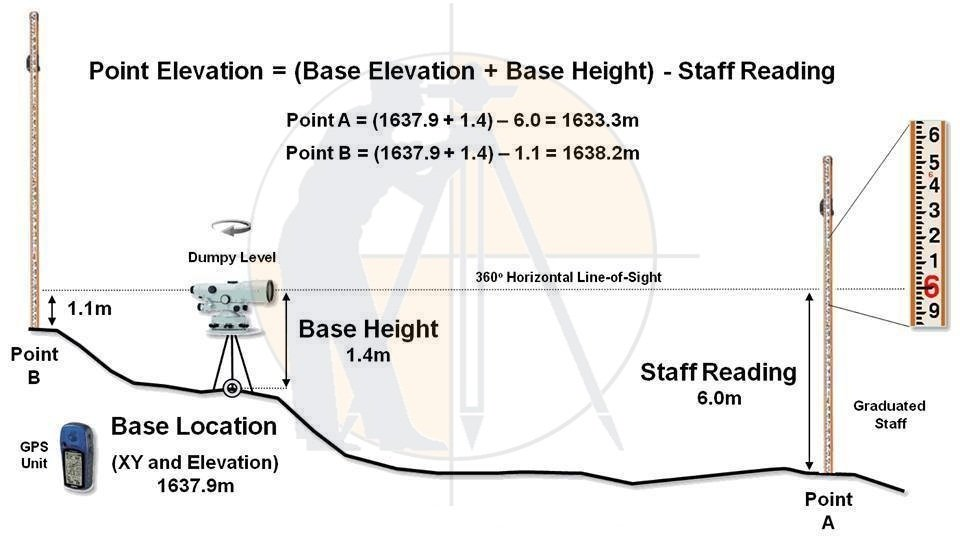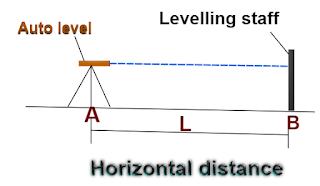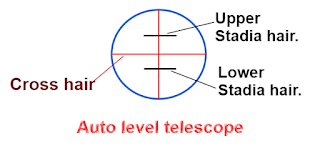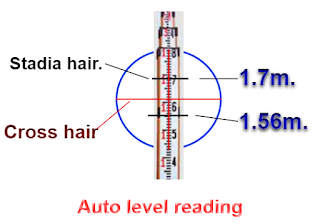Breaking News
Home / Land Surveying / How To Calculate Horizontal Distance Using With Auto Level Machine

# How To Calculate Horizontal Distance Using With Auto Level Machine

### How To Calculate The Horizontal Distance Using With Auto Level Machine

Now, let us go through the procedure so that we can calculate the horizontal distance between the two points, by only using an auto-level machine & leveling staff.As you can observe in the above drawing, we will find out the distance L between points A & B, without using the measuring tape.

### Procedure:

1. Set up the tripod legs & fix the auto level to the tripod.

2. Level the auto level machine by centering the bubble in a vial.

3. Transfer the auto-level position over the ground by plumb bob & let us name it point A.

4. Hold the staff vertically at point B.

5. Adjust the telescope lens until you observe the cross-hair & stadia hair clearly.As you can observe in the above drawing, the telescope consists of two stadia hairs i.e. upper & lower stadia hair.

6. Focus the telescope over the leveling staff, positioned over point B.

7. Take the 2 readings of the staff at the upper & lower stadia hair level as shown below.### Now, the horizontal distance L

= [( 1.7m. – 1.56m ) × 100]

= [ 0.14m.× 100]

14m.

### Note:

In the auto level machine, the difference in the stadia hair reading is set at the 1: 100 ratio. i.e. if the reading difference is 1cm, that represents 1m.over the ground.

So, to get the measurement over the ground, you have to multiply the difference in the stadia hair readings by 100.

### THANKS.